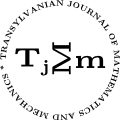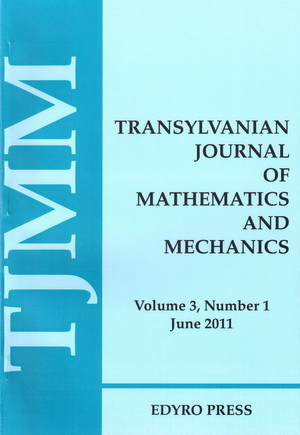ISSN: 2067-239X
ISSN(on-line): 2067-239X

Indexed in:
Mathematical Reviews
Zentralblatt MATH

Front cover# Volume 7 (2015), Number 1

## Univalence Criteria for Analytic Functions Deﬁned by Certain Generalized Differential Operator

### Author(s): ROBERTA BUCUR

Abstract: In this paper, we consider the generalized differential operator ${D}_{\alpha ,\mu }^{n}\left(\lambda ,\omega \right)f\left(z\right)$ and we determine some sufficient conditions for univalence of analytic functions deﬁned by this operator. Relevant connections of some results obtained with those in earlier works are also given.

## New Integral Solutions for a Thermoelastic Quarter-plane

### Author(s): ION CREȚU

Abstract: In this paper new inﬂuence functions on the thermal displacements and stresses of a unit point heat source for a boundary value problems of thermoelasticity for a quarter-plane were obtained. Also, new integral solutions of Green’s for a boundary value problems were derived, thermal stresses caused by the temperature gradient acting on a segment of the boundary line were calculated. All these results are presented in terms of elementary functions for canonical domains of Cartesian system coordinates. Using the computer program Maple 18, the graphical presentations of thermal stresses caused by a unit point of heat source and of thermal stresses for one boundary value problems caused by the temperature gradient acting on a segment of the boundary line were constructed.

## An Integral Equation from Physics - A Synthesis Survey - Part I

### Author(s): MARIA DOBRIȚOIU

Abstract: In this synthesis survey we will present in several parts, the results obtained by the author in the study of the integral equation from physics: $x\left(t\right)={\int }_{a}^{b}K\left(t,s,x\left(s\right),x\left(a\right),x\left(b\right)\right)ds+f\left(t\right),\phantom{\rule{1em}{0ex}}t\in \left[a,b\right].$ that appeared in the 70s and was a mathematical model in the operation of turbojets. This is the ﬁrst part of the synthesis survey and it contains the results concerning the existence and respectively the existence and uniqueness of the solution of this integral equation. Also it contains some properties of the solution of this integral equation, formulated as integral inequalities. This part ends with three examples.

## Some Perturbed Ostrowski Type Inequalities for Absolutely Continuous Functions (III)

### Author(s): S.S. DRAGOMIR

Abstract: In this paper, some new perturbed Ostrowski type inequalities for absolutely continuous functions are established.

## On Relative Order Oriented Results of Entire Functions of Two Complex Variables

### Author(s): SANJIB KUMAR DATTA, TANMAY BISWAS and GOLOK KUMAR MONDAL

Abstract: In this paper we intend to ﬁnd out relative order (relative lower order) of an entire function of two complex variables $f$ with respect to another entire function of two complex variables $g$ when relative order (relative lower order) of $f$ and relative order (relative lower order) of $g$ with respect to another entire function $h$ of two complex variables are given.

## The Order of Convexity for an Integral Operator

Abstract: For analytic functions ${f}_{i}$, ${g}_{i}$ for $i=1,2,..,n$, $n\in {ℕ}^{\ast }$, in the open unit disk $\mathsc{𝒰}$, we study some convexity properties for a new general integral operator.

## Some Inequalities for the Growth of Self-reciprocal Polynomials

### Author(s): Q.M. TARIQ

Abstract: In this paper we prove a property of self reciprocal polynomials and use it to obtain few inequalities for the growth of such polynomials.

## Oscillation of Second Order Quasilinear Difference Equations with Several Neutral Terms

### Author(s): E. THANDAPANI, D. SEGHAR and S. SELVARANGAM

Abstract: This paper deals with the oscillatory properties of a certain second order difference equation with several neutral terms. Some new sufficient conditions are established which simplify the examination of the equation studied. The results obtained here extend those in  for second order difference equation.

## On Čebyšev-Grüss Type Inequalities for Double Integrals

### Author(s): MEHMET ZEKI SARIKAYA and MEHMET EYUP KIRIS

Abstract: In this study, we establish some new inequalities of Čebyšev-Grüss type involving functions of two independent variables for double integrals. The analysis used in the proofs is elementary and our results provide new estimates on inequalities of this type.